## Preliminaries

Before you begin learning, relearning, or reviewing algebra, you need to feel comfortable with some pre-algebra terms and operations. The first items you should become familiar with are the different categories or types of numbers and the common math symbols.

#### Categories of numbers

In doing algebra, you work with several categories of numbers.

• Natural or counting numbers. The numbers 1, 2, 3, 4, …are called natural or counting numbers.

• Whole numbers. The numbers 0, 1, 2, 3, …are called whole numbers.

• Integers. The numbers …–2, –1, 0, 1, 2, …are called integers.

• Negative integers. The numbers …–3, –2, –1 are called negative integers.

• Positive integers. The natural numbers are sometimes called the positive integers.

• Rational numbers. Fractions, such as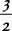or, are called rational numbers. Since a number such as 5 may be written as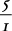, all integers are rational numbers. All rational numbers can be written as fractions, with a being an integer and b being a natural number. Terminating and repeating decimals are also rational numbers, because they can be written as fractions in this form.

• Irrational numbers. Another type of number is an irrational number. Irrational numbers cannot be written as fractions, with a being an integer and b being a natural number.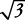and π are examples of irrational numbers. An irrational number, when exactly expressed as a decimal, neither terminates nor has a repeating decimal pattern.

• Even numbers. Even numbers are integers divisible by 2: … –6, –4, –2, 0, 2, 4, 6, …

• Prime numbers. A prime number is a natural number that has exactly two different factors, or that can be perfectly divided by only itself and 1. For example, 19 is a prime number because it can be perfectly divided by only 19 and 1, but 21 is not a prime number because 21 can be perfectly divided by other numbers (3 and 7). The only even prime number is 2; thereafter, any even number may be divided perfectly by 2. Zero and 1 are not prime numbers or composite numbers. The first 10 prime numbers are 2, 3, 5, 7, 11, 13, 17, 19, 23, and 29.

• Odd numbers. Odd numbers are integers not divisible by 2: …–5, –3, –1, 1, 3, 5,….

• Composite numbers. A composite number is a natural number divisible by more than just 1 and itself: …4, 6, 8, 9,…

• Squares. Squares are the result when numbers are multiplied by themselves, that is, raised to the second power. 2 · 2 = 4; 3 · 3 = 9. The first six squares of natural numbers are 1, 4, 9, 16, 25, 36.

• Cubes. Cubes are the result when numbers are multiplied by themselves and then again by the original number, that is, raised to the third power. 2 · 2 · 2 = 8; 3 · 3 · 3 = 27. The first six cubes of natural numbers are 1, 8, 27, 64, 125, 216.

#### Ways to show multiplication

There are several ways to show multiplication of a pair of numerical values.

• When the two numerical values are known, you can show the multiplication of 4 with 3 as follows: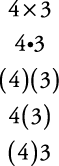• When one value is a number and the other value is a variable: show multiplication of 4 and a as follows:• When both values are variables: show the multiplication of a and b as follows: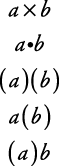#### Common math symbols

The following math symbols appear throughout algebra. Be sure to know what each symbol represents.

Symbol references:

• = is equal to

• ≠ is not equal to

• > is greater than

• < is less than

• ≥ is greater than or equal to (also written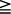)

• ≤ is less than or equal to (also written)

•is not greater than

•is not less than

•is not greater than or equal to

•is not less than or equal to

• ≈ is approximately equal to (also)